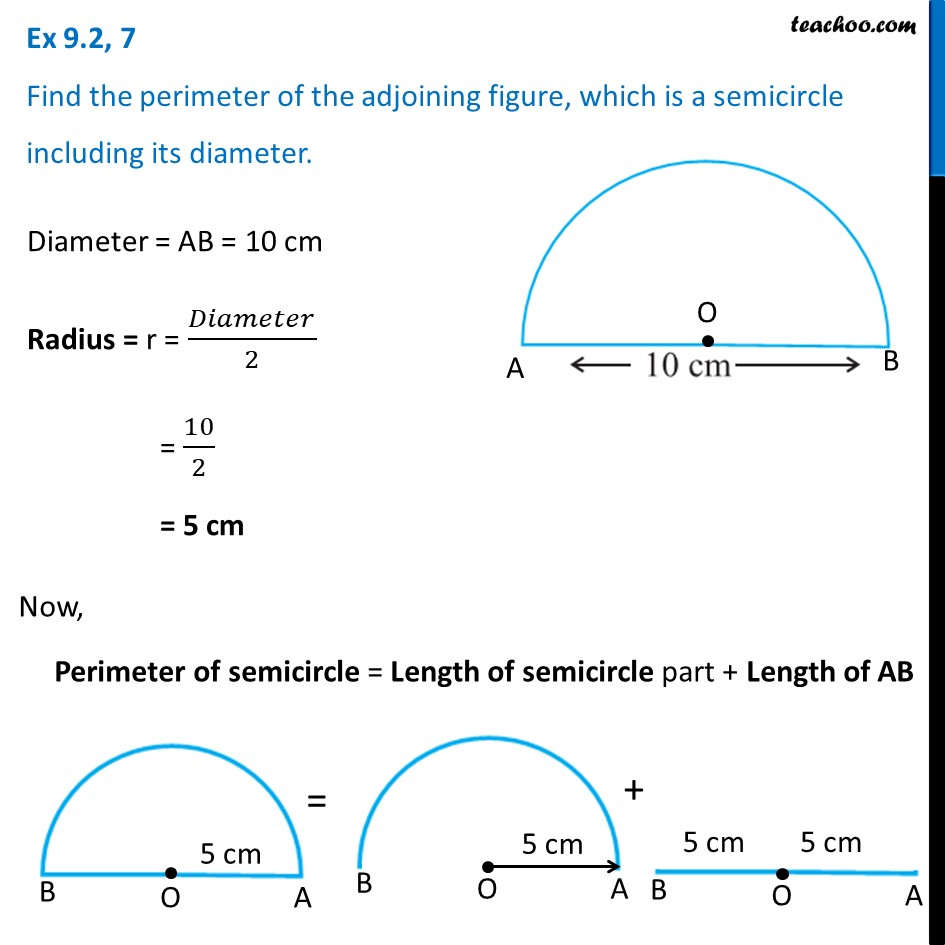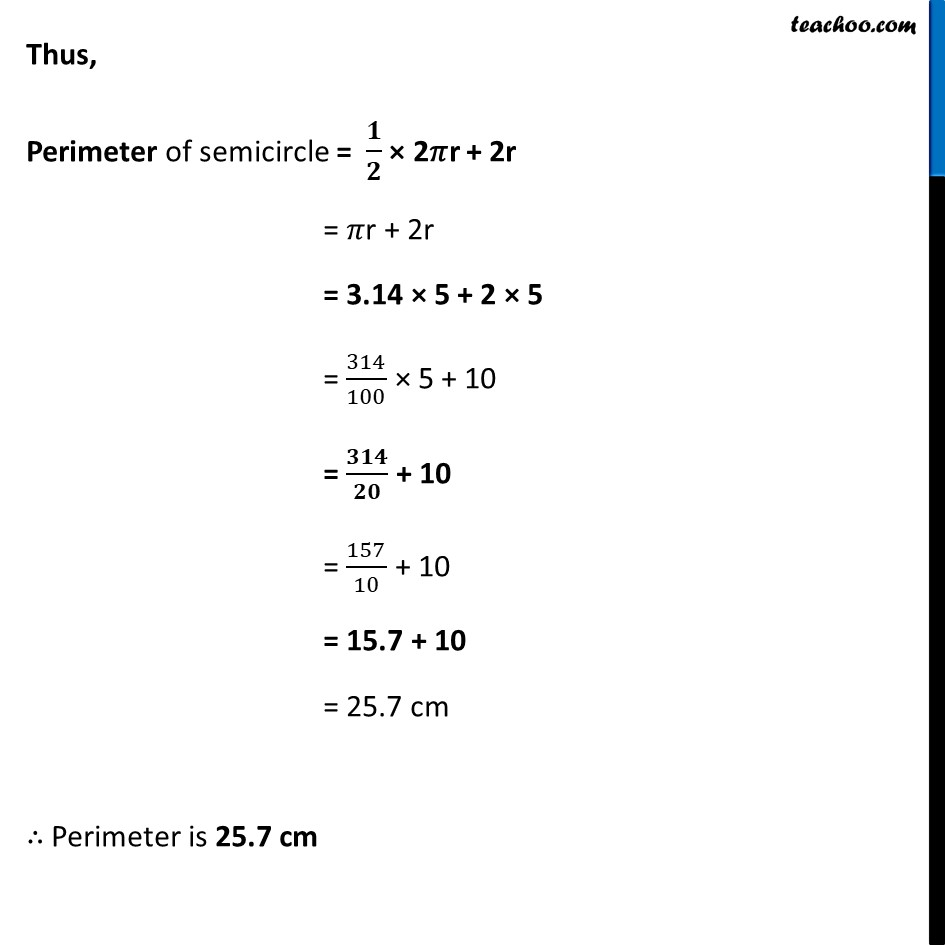Ex 9.2

Chapter 9 Class 7 Perimeter and Area
Serial order wiseLearn in your speed, with individual attention - Teachoo Maths 1-on-1 Class

### Transcript

Ex 9.2, 7 Find the perimeter of the adjoining figure, which is a semicircle including its diameter. Diameter = AB = 10 cm Radius = r = 𝐷𝑖𝑎𝑚𝑒𝑡𝑒𝑟/2 = 10/2 = 5 cm Now, Perimeter of semicircle = Length of semicircle part + Length of AB Thus, Perimeter of semicircle = 𝟏/𝟐 × 2𝜋r + 2r = 𝜋r + 2r = 3.14 × 5 + 2 × 5 = 314/100 × 5 + 10 = 𝟑𝟏𝟒/𝟐𝟎 + 10 = 157/10 + 10 = 15.7 + 10 = 25.7 cm ∴ Perimeter is 25.7 cm